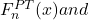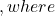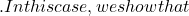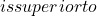# Two new estimators of distribution functions

This paper considers the estimation of a distribution functionbased on
a random samplewhen the sample is suspected to come from a
close-by distribution. Two new estimators, namelyF^{S}_n(x)F_n(x)F_X(x) = F_0(x) + n^{-1/2}\delta\mbox{max}_x |F_X(x) − F_0(x)| \leq n^{-1/2} \deltaF^S_n (x)F^{PT}_n (x)F_0(x)\$.

Fulltext